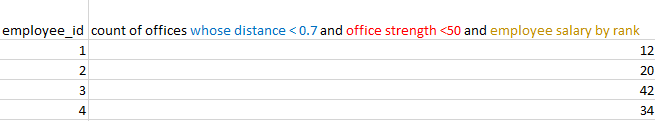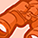# QlikView App Dev

Discussion Board for collaboration related to QlikView App Development.

Announcements
Now Live: Qlik Sense SaaS Simplified Authoring – Analytics Creation for Everyone: READ DETAILS
cancel
Showing results for
Did you mean:Creator III

## Haversine formula to find distance between two lat/long points

I figured I would take a moment to share a code snippet that uses the Haversine formula to calculate the distance between two lat/long points.

`// use the 'haversine' formula to calculate distance between two lat/long points for each record// The Haversine formula can be broken into multiple pieces or steps - I chose to do the entire formula// in one step. This makes it more difficult to read/understand, but allows QlikView to do it all at once// when reading a record. If I broke it up, then QlikView would have to examine each record for each step of the// process increasing time and decreasing efficiency.// formula notes:// http://www.movable-type.co.uk/scripts/latlong.html// convert degrees to radians: rads=degrees*pie / 180// radius of earth = 6371km (constant)CalcDistances:noconcatenateload zipcode, state, country, Latitude1, Longitude1, weatherStationID, Latitude2, Longitude2, // the Haversine formula (2 * (atan2( sqrt((sqr(sin(((Latitude2 * Pi() / 180) - (Latitude1 * Pi() / 180)) / 2)) + ((cos((Latitude1 * Pi() / 180)) * cos((Latitude2 * Pi() / 180))) * sqr(sin(((Longitude2 * Pi() / 180) - (Longitude1 * Pi() / 180)) / 2)) ) )), sqrt((1-( sqr(sin(((Latitude2 * Pi() / 180) - (Latitude1 * Pi() / 180)) / 2)) + ((cos((Latitude1 * Pi() / 180)) * cos((Latitude2 * Pi() / 180))) * sqr(sin(((Longitude2 * Pi() / 180) - (Longitude1 * Pi() / 180)) / 2)) ) )) )))) * 6371 as Distance_kmresident AllPossibleZipWeatherStationCombinations;`

Example of input/output:- Trevor

19 RepliesChampion III

Not correct, and yes we can calculate using two tables. Easiest way is to just move most of the calculation to the front end, but if performance becomes a problem, there are other ways to handle it. See attached.Not applicable

Thank you so much John again you solved my problemNot applicable

Hi John,

I'm struggling with an issue john, the first thought in my mind is you, who can help me out. who can help in any issue

i have  data as below

based on your inputs i have calculated distance between two latitude and longitudes

1, i need to calculate the distance between each employee to every office (this is achieved with your previous inputs)

the main part is

i want three input boxes  as below

when i put value in  distance inputbox  =  0.7 all the offices which are less than 0.7 km  to employee should  be dispalyed

when i put value in  office strength inputbox  = 100 all the offices stregth which are less than 100  should  be dispalyed

when i put value in    employee salary rank inputbox    = 15  top 15 ranked employees should be displayed

all the above should be displayed in a straight table

as below

output_1

employee  emp_lat  emp_lang                  office strength                                    employee salary        count(offices <0.7)

1                  x            y                    50(which is less than 100 )                                10 (rank 1)            12(varies)

2                  z            w                  40(which is less than 100 )                                8 (rank 2)              1

i want for a particular employee how many offices are below 0.7 km(what ever the value provided in input box),  whose office strength  is less than 100(what ever the value provided in inputbox) and the employee salary by rank (provided in the input box)Champion III

Calculate distance as shown before. Add a Rank field for the salary. Trigger actions to make selections based off the input boxes. See attached.Not applicable

Hi John Thank you for the reply

my requiremnt is  a staright table

as below

based on the input boxes valuesi have defined two variables and a set expression as belowi'm using  emp_id as dimension

this is my expression in staraight table

count({<Distance={"<\$(vdistance_office)"}, office_strength={"<\$(v_strength)"}>}office_id)

i'm not getting the exat results and my data is associated as belowChampion III

Well, that's very different from the approach I took, but I think it should work just fine. You should count distinct office_id, not just office_id, but I don't know if that's the problem. Hard to debug what I can't see.Contributor III

Hi,

the formula runs without errors, but it doesn't calculate the measure.

Which format for longitude lattitude is necessary?

BR

IngoContributor III

Heres a screenshot of my dataContributor II

Hello Chong Ee Lian,

I could not understand what you have done on introduce an additional recnum() column for the table in the data load editor.Creator II

Hi, this is an old thread, but I needed a solution and have written some code using the Vicenty formula if anyone finds its useful.  I stole @johnw 's code above.

For ease of understanding (and copy and paste), I broke the QlikView Load statement into 3 preceding loads:

``````Trace Calculating distance on a sphere;

// This is a series of preceding loads, executed in the order Step 1... Step 2...
NoConcatenate
Distances:
// Step 3: Convert the raw distance into convenient common units
*,

Round ( [Distance Vicenty], 0.1 ) 				as [Distance Vicenty km],
Round ( [Distance Vicenty] * 1000 ) 			as [Distance Vicenty meters],
Round ( [Distance Vicenty] * 0.6213712, 0.1 ) 	as [Distance Vicenty miles],
;
// Step 2: Calculate the distance using the Vicenty formula and radian fields
*,

// Vincenty formula
atan2(
sqrt(
+
),
+
) * 6371 as [Distance Vicenty]

;
// Step 1: Generate radian fields
// Replace Lat1, Lat2, Lon1 and Lon2 with the name of your fields
*,
(Lat1 * Pi() / 180)	as Lat1Radians,
(Lat2 * Pi() / 180)	as Lat2Radians,
(Lon1 * Pi() / 180)	as Lon1Radians,
(Lon2 * Pi() / 180)	as Lon2Radians
Resident TestLocations
;``````

This results in some extra fields but they can be dropped easily and this is much easier to understand.  I have four actual places as test data and the QVW attached calculates the Vicenty distance to the same end location (Salt Lake City airport).

The file DistanceCalculations.qvw is attached.